Courses

# Linear Algebra NAT Level - 2

## 10 Questions MCQ Test Topic wise Tests for IIT JAM Physics | Linear Algebra NAT Level - 2

Description
This mock test of Linear Algebra NAT Level - 2 for Physics helps you for every Physics entrance exam. This contains 10 Multiple Choice Questions for Physics Linear Algebra NAT Level - 2 (mcq) to study with solutions a complete question bank. The solved questions answers in this Linear Algebra NAT Level - 2 quiz give you a good mix of easy questions and tough questions. Physics students definitely take this Linear Algebra NAT Level - 2 exercise for a better result in the exam. You can find other Linear Algebra NAT Level - 2 extra questions, long questions & short questions for Physics on EduRev as well by searching above.
*Answer can only contain numeric values
QUESTION: 1

### If (a, 6) are the values for which the given equations are inconsistent. x + 2y + 3z = 4 x + 3y + 4z = 5 x + 3y + az = b then the value of a is :

Solution:

Consider the augmented matrix,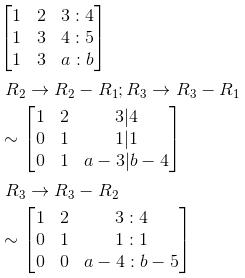For the following to be inconsistent, a – 4 = 0  and  b – 5 ≠ 0
⇒  a = 4  and  b ≠ 5

The correct answer is: 4

*Answer can only contain numeric values
QUESTION: 2

### If  z = 7c, then find the value of x such that the given equations has infinite solution x + 3y – 2z = 0 2x – y + 4z = 0 x – 11y + 14z = 0

Solution:

The given system of equations is equivalent to the single matrix equation, AX = 0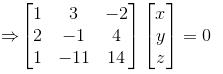To get the solution, consider the augmented matrix,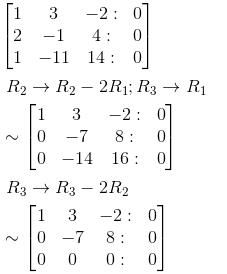⇒ z  is a free variable. The given system reduces to
x + 3y – 2z = 0
–7x + 8z = 0
Let  z = c,  where  is an arbitrary number. Then,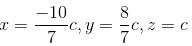If we take c = 7, we get the solution as  (–10  8  7)T

The correct answer is: -10

*Answer can only contain numeric values
QUESTION: 3

### A matrix M has eigenvalues 1 and 4 with corresponding eigenvectors (1, –1)T and (2, 1)T respectively. Then M  is given by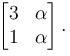Find the value of α

Solution:

As the given eigen vectors of M  are linearly independent, M  is diagonalisable,
∴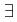matrices P and D  such that,
or  D = P–1MP
M = PDP–1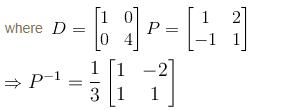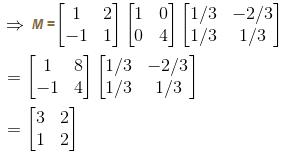The correct answer is: 2

*Answer can only contain numeric values
QUESTION: 4

The only real value of λ for which the following equations have non-zero solution is,
x + 3y + 3z = λx
3x + y + 2z = λy
2x + 3y + z = λz

Solution:

The given system is,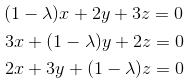For the given system of equations to have a non-zero solution, the coefficient matrix, say A, must be of rank less than 3. Thereby, det A must be zero i.e.,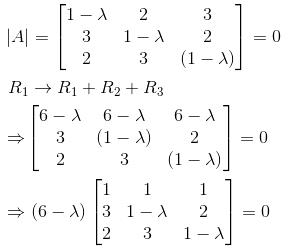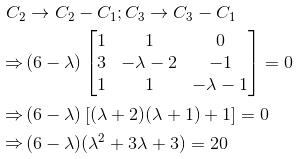The roots of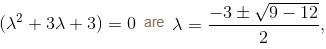are imaginary.
Hence the only real value of λ  for which the system of equations will have a non-zero solution is 6.

The correct answer is: 6

*Answer can only contain numeric values
QUESTION: 5

For what value of c the following given equations will have infinite number of solutions?
–2x + y + z = 1
x – 2y + z = 1
x + y – 2z = c

Solution:

Consider the augmented matrix,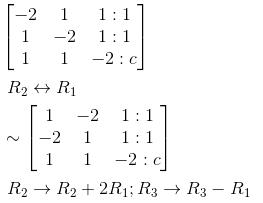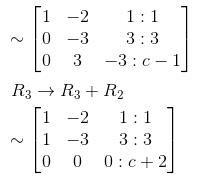The system will have infinite number of solution if  c = –2.

The correct answer is: -2

*Answer can only contain numeric values
QUESTION: 6

Find the rank of the matrix for the following equations :
3x + 4y – z – 6w = 0
2x + 3y + 2z – 3w = 0
2x + y – 14z – 9w = 0
x + 3y + 13z + 3w = 0

Solution:

Let A  be the coefficient matrix, i.e., consider,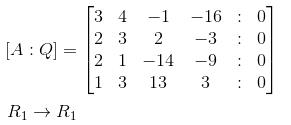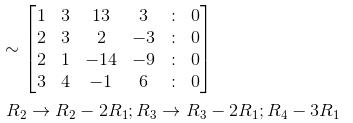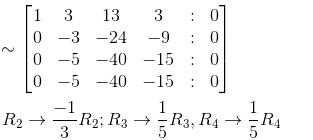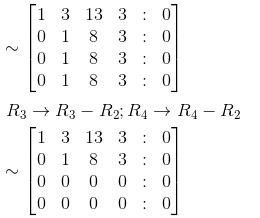∴  The rank of the matrix is 2.

The correct answer is: 2

*Answer can only contain numeric values
QUESTION: 7

Let A  be a 3 × 3 matrix. Suppose that the eigenvalues of A are –1, 0, 1 with respective eigenvectors (1,–1,0)T, (1,1,–2)T & (1,1,1)T. Then 6A is given by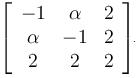Find the value of  α

Solution:

We know that,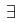the matrices D and P such that D = P–1AP is a diagonal matrix.

In this case,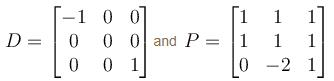∵  D = P–1AP

Pre multiplying by P  and post multiplying by P–1, we get  A = PDP–1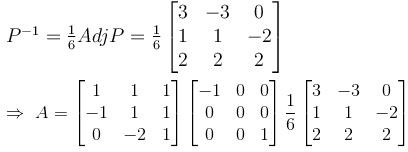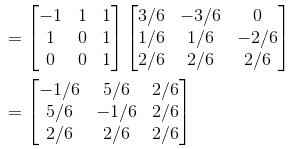Hence,   6A =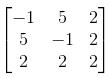The correct answer is: 5

*Answer can only contain numeric values
QUESTION: 8

Find the rank of the matrix for the following equations :
4x + 2y + z + 3u = 0
6x + 3y + 4z + 7u = 0
2x + y + u = 0

Solution:

Consider the augmented matrix, [A : O]  where A the coefficient matrix,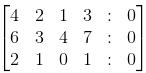interchanging the variables x and z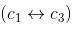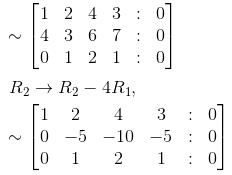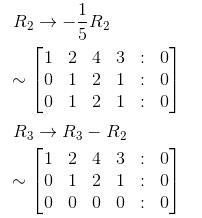∴   The rank of the matrix is 2.

The correct answer is: 2

*Answer can only contain numeric values
QUESTION: 9

Solution of the system,
3x – 2y – w = 2
2y + 2z + w = 1
x – 2y – 3z + 2w = 3
y + 2z + w = 1
is given by (1, 0, 0, α)T. Find the value of α.

Solution:

Consider the augmented matrix,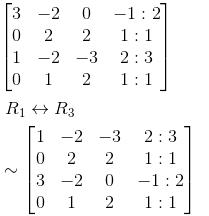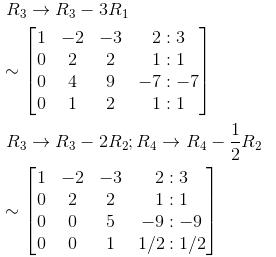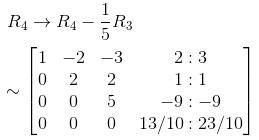So, the given system reduces to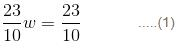5z – 9w = –9          ....(2)
2y + 2z + w = 1     ....(3)
x – 2y – 3z + 2w = 3 ....(4)
from (1) we get, w = 1
from (2) we get, z = 0
from (3) we get, y = 0
from (4) we get, x = 1

The correct answer is: 1

*Answer can only contain numeric values
QUESTION: 10

Let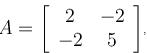a real symmetric matrix. P is the orthogonal matrix such that P-1 AP is a diagonal matrix given by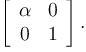Find the value of  α

Solution: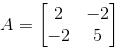tr(A) = 7 ; |A| = 6
∴ The characteristic equation will be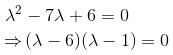⇒ λ = 6, 1 are the eigenvalues of  A.
Now, Let (xy) be the eigenvector corresponding to λ = 6, then,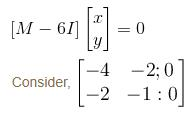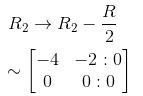i.e., –4x – 2y = 0
or  2x + y = 0
A non zero solution is  u1 = (1, –2)
Now, again if (xy) is the eigenvector corresponding to λ = 1. Then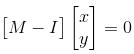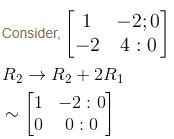i.e., x – 2y = 0

A non-zero solution is  u2 = (2, 1)
Now, u1 and u2 will be orthogonal. Normalising u1and u2 yield the orthonormal vectors,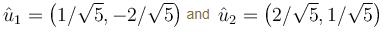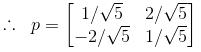such that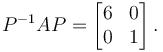The correct answer is: 6